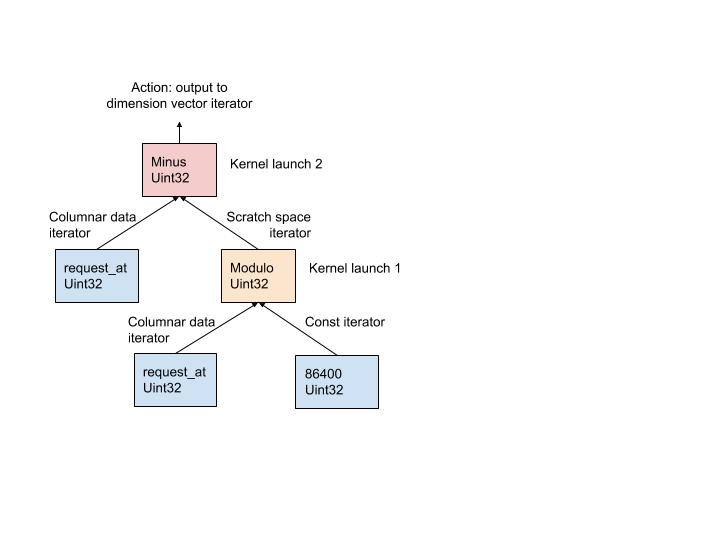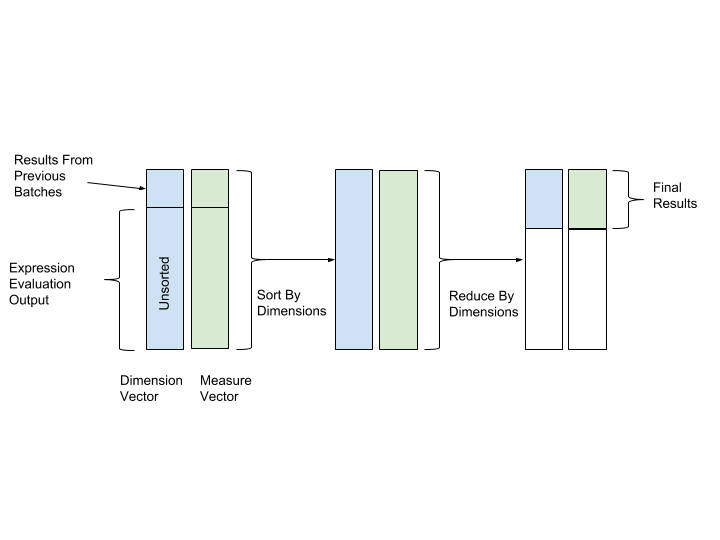# Query Execution

AresDB utilize the Thrust library to implement query execution procedures for simplicity, which offers fine-tuned parallel algorithm building blocks for quick implementation in the current query engine.

In Thrust, input and output vector data is accessed using random access iterators. Each GPU thread seeks the input iterators to its workload position, reads the values and performs the computation, and then writes the result to the corresponding position on the output iterator.

AresDB follows the one-operator-per-kernel (OOPK) model for evaluating expressions. The following diagram demonstrates this procedure on an example AST, generated from a dimension expression request_at - request_at % 86400 in the query compilation stage.Figure: One-operator-per-kernel model for expression evaluation

In Figure above, traversing each leaf node returns an iterator for its parent node. In cases where the root node is also a leaf, the root action is taken directly on the input iterator.

At each non-root non-leaf node (modulo operation in this example), a temporary scratch space vector is allocated to store the intermediate `(request_at % 86400)` results for output. Leveraging Thrust, a kernel function is launched to compute the output for this operator on GPU. The results are stored in the scratch space iterator.

At the root node, a kernel function is launched in the same manner as a non-root, non-leaf node. Different output actions are taken based on the expression type, detailed below:

• Filter action to reduce the cardinality of input vectors
• Write dimension output to the dimension vector
• Write measure output to the measure vector

Sorting and Reduction are then executed after expression evaluation to conduct final aggregation. In both sorting and reduction operations, we use the values of dimension vector as key of sorting and reduction, and the values of measure vector as the values to aggregate on. In this way, rows with same dimension values will be grouped together and aggregated. Figure 12 depicted the sorting and reduction process below:Figure: Sort and reduce by key on dimension (key) and measure (value) vector

AresDB also supports the following advanced query features:

• Join: currently AresDB supports hash join from fact table to dimension table
• Hyperloglog Cardinality Estimation
• Geo Intersect: currently AresDB supports only intersect operations between GeoPoint and GeoShape

Home